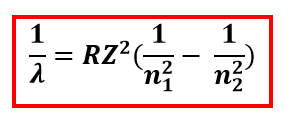# Problem: Why are the emission wavelengths for hydrogen and helium different? And why does the equation that relates to emission spectra to the energy levels differ for hydrogen and helium?

###### FREE Expert Solution

The Rydberg equation relates the emission wavelength to the energy levels:where

80% (197 ratings)###### Problem Details

Why are the emission wavelengths for hydrogen and helium different? And why does the equation that relates to emission spectra to the energy levels differ for hydrogen and helium?